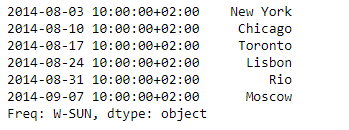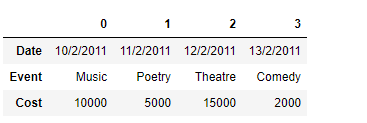GeeksforGeeks App
Open AppBrowser
Continue

# Python | Pandas Series.transpose()

Pandas series is a One-dimensional ndarray with axis labels. The labels need not be unique but must be a hashable type. The object supports both integer- and label-based indexing and provides a host of methods for performing operations involving the index.

Pandas` Series.transpose()` function return the transpose, which is by definition self.

Syntax: Series.transpose(*args, **kwargs)

Parameter : None

Returns : self

Example #1: Use `Series.transpose()` function to find the transpose of the given Series object.

 `# importing pandas as pd``import` `pandas as pd`` ` `# Creating the Series``sr ``=` `pd.Series([``'New York'``, ``'Chicago'``, ``'Toronto'``, ``'Lisbon'``, ``'Rio'``, ``'Moscow'``])`` ` `# Create the Datetime Index``didx ``=` `pd.DatetimeIndex(start ``=``'2014-08-01 10:00'``, freq ``=``'W'``, ``                     ``periods ``=` `6``, tz ``=` `'Europe/Berlin'``) `` ` `# set the index``sr.index ``=` `didx`` ` `# Print the series``print``(sr)`

Output :Now we will use `Series.transpose()` function to find the transpose of the given series object.

 `# find the transpose``sr.transpose()`

Output :As we can see in the output, the `Series.transpose()` function has returned the same object as the transpose of the given series object, which is by definition self.

Example #2: Use `Dataframe.transpose()` function to find the transpose of the given Dataframe.

 `# importing pandas as pd``import` `pandas as pd`` ` `# Creating the Dataframe``df ``=` `pd.DataFrame({``'Date'``:[``'10/2/2011'``, ``'11/2/2011'``, ``'12/2/2011'``, ``'13/2/2011'``],``                    ``'Event'``:[``'Music'``, ``'Poetry'``, ``'Theatre'``, ``'Comedy'``],``                    ``'Cost'``:[``10000``, ``5000``, ``15000``, ``2000``]})`` ` `# Print the dataframe``print``(df)`

Output :Now we will use `Dataframe.transpose()` function to find the transpose of the given Dataframe.

 `# find the transpose``df.transpose()`

Output :As we can see in the output, the `Dataframe.transpose()` function has successfully returned the transpose of the given Dataframe object.

My Personal Notes arrow_drop_up